# Cost function and process of learning in Machine Learning

Machine Learning and Artificial Intelligence techniques try to mimic human intelligence. But have we ever thought,

how do people decide that now it has started mimicking the human and earlier it was not?

#### Key takeaways from this blog

1. The basic intuition behind cost function and its importance in Machine Learning.
2. A step-wise learning process for Machine Learning algorithms.
3. How machine stores its learnings?
4. What are contour plots?

In this article, we will discuss how we say that "machine has achieved intelligence"? Most of us must be thinking that, Isn't it when machines stop making errors or mistakes?

Yes! Precisely in the same manner. But this process is quite rigorous yet straightforward to understand.

But how can a non-living thing achieve the status of "Intelligent"?

In its earlier stages, it might be making some mistakes and learning from several experiences. But how? Let’s move towards finding the answer to this question. Let's take one data set and visualize the learning steps for machines.

In machine learning, machines learn the mapping function, which maps input data to the output data. But what exactly it means to learn a function?

Suppose we have input and output data from a straight line equation, Y = 2*X. We can also say Y = 2X* is the function that machines are expected to learn. Let's represent it in X, Y coordinate form for all samples,

``````(X,Y) = [(0,0), (1,2), (2,4), (3,6), (4, 8), ..., (50, 100)]
X = [0, 1, 2, ..., 50]
Y = [0, 2, 4, ..., 100]
#There are 51 samples. How? (Think!)
``````

If we represent these (X, Y) points in the cartesian 2D plane, then the curve will be similar to what is shown in the image below.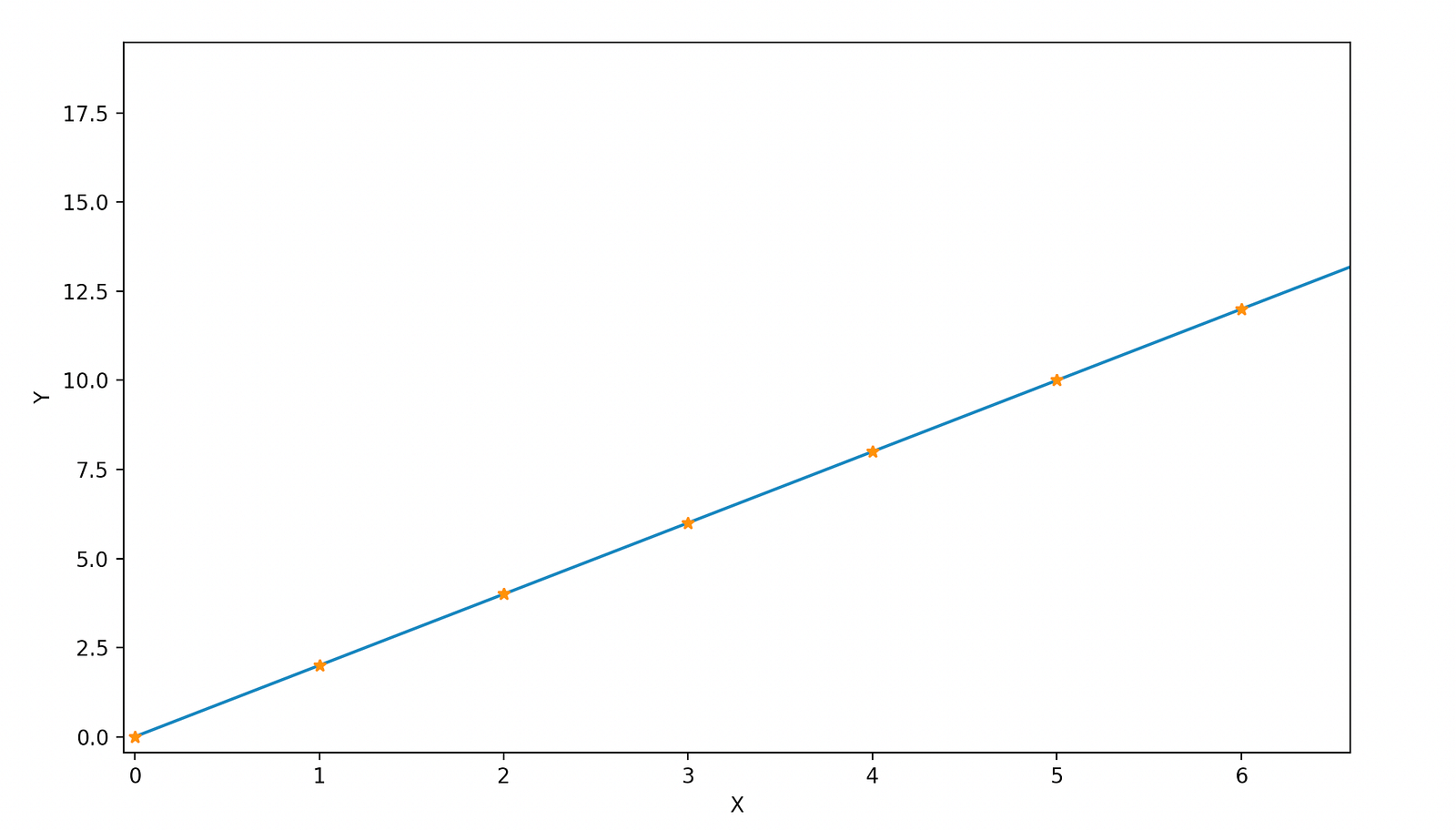Now, suppose we need the machine to learn a "linear" function of the form,In simple terms, our job is done if the machine can find the perfect value of this θ1 (which we know as θ1 = 2). Right?

But how this will be done?

Let's explore the process of this learning in simple steps.

#### Step 1

The machine will choose a random value for θ1. Suppose our model picked θ1 = 1. Based on this parameter, the machine will calculate the Y', which is different from Y, and calculated from the Y' equation, Y’ = 1*X.

``````X = [0, 1, 2, ..., 50]
Y' = [0, 1, 2, ..., 50]
``````

#### Step 2

Now the machine has 2 Ys, one is Y, and another is Y'. But it knows that Y is an accurate one and Y' is the estimated one. So now, it will calculate the error between the estimated Ys (Y') and the actual Ys (Y) to sense by how much the initial guess of θ1 was wrong.
Let's define this error as a simple difference between these two Ys.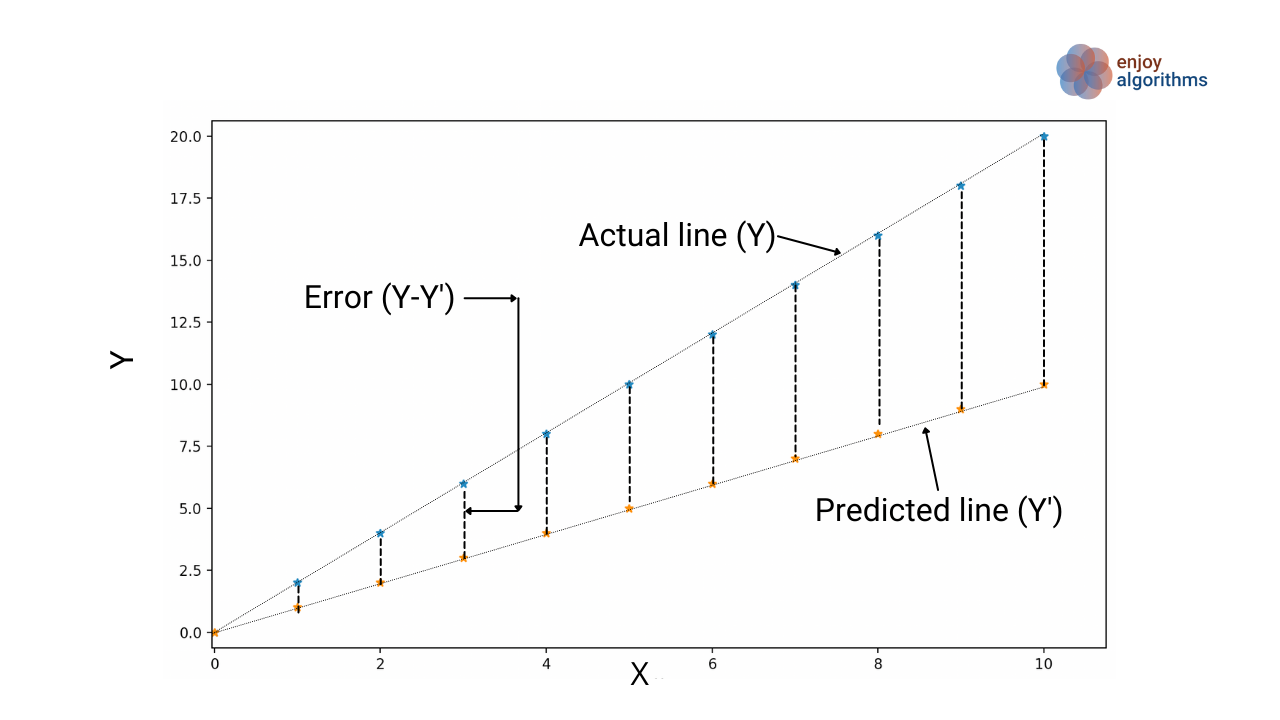We have 51 data samples, so to take account of all the samples, we define an average error over all the data samples.Always keep in mind that our objective is to decrease this error as minimum as possible. From this objective, we can also say that our "average error" is similar to some "Cost" functions. Our goal is to minimize the cost.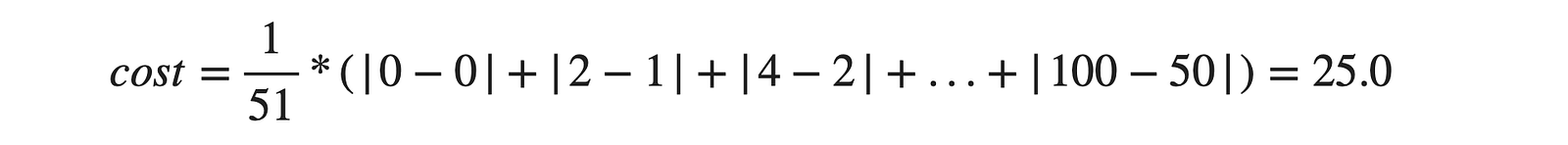#### Step 3

In the next run, the machine will update the value of θ1 so that this average error gets reduced. Suppose we plot the average error or cost function with respect to various values of θ1 that our machine is guessing or choosing randomly. Then we will get a curve, something like shown in the below plot.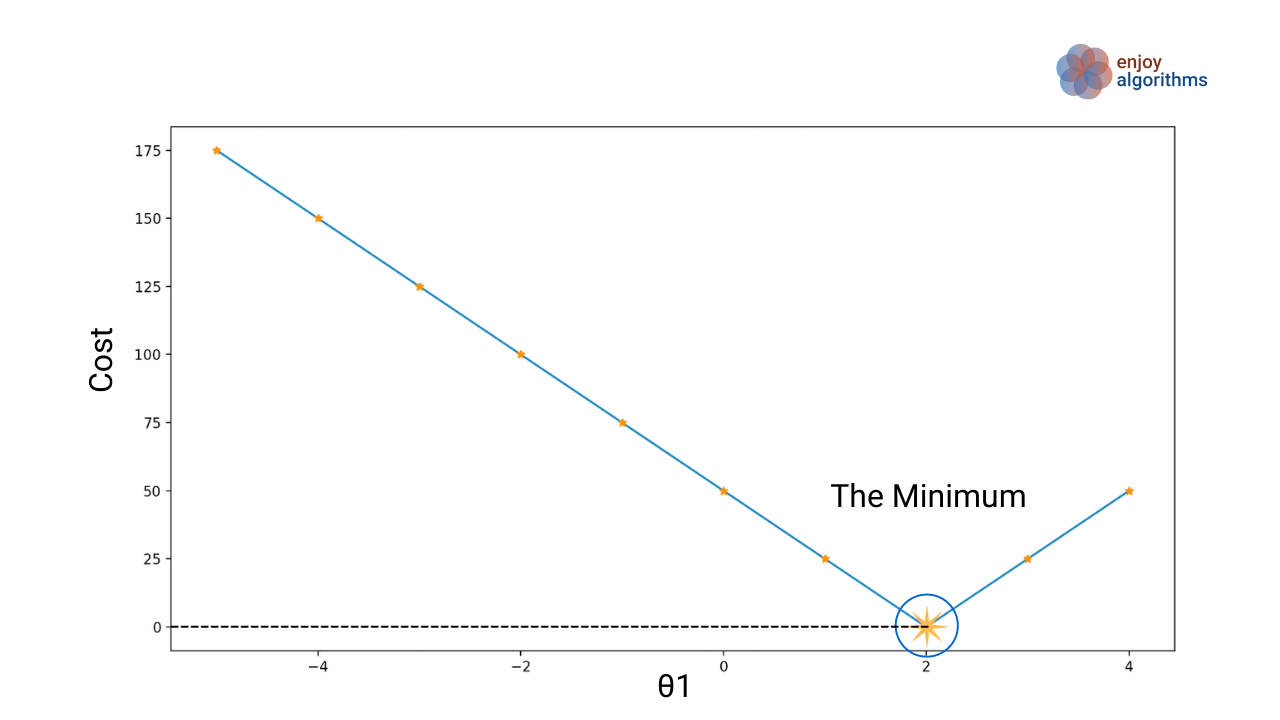If we remember correctly, our objective was to minimize the cost function. So if we have to choose the value for θ1 for which the cost has a minimum value, we would definitely select θ1 = 2. Right?

#### Step 4

Now the machine knows that for θ1 = 2, the error/cost function is minimum. So it will store this value of θ1in the memory, and we will express this phenomenon as,

“Machine has learned!!”

#### Step 5

Again, for any new value of X that was not seen earlier by the machine, let's say X = 500. It will just take the input of X and give the result as θ1*X, i.e., 2*500 = 1000.

Hurrah! That’s how Machine learns in Machine Learning!!

Note: Please remember that we have solved a fundamental problem statement where we had to learn just one parameter, which we estimated based on minimizing the cost function.

### Taking an Example of 2 parameter learning

From our fundamental knowledge of mathematics, we know that the straight-line equation seems to be in the form ofWhere θ1 corresponds to slope and θ0 is the intercept.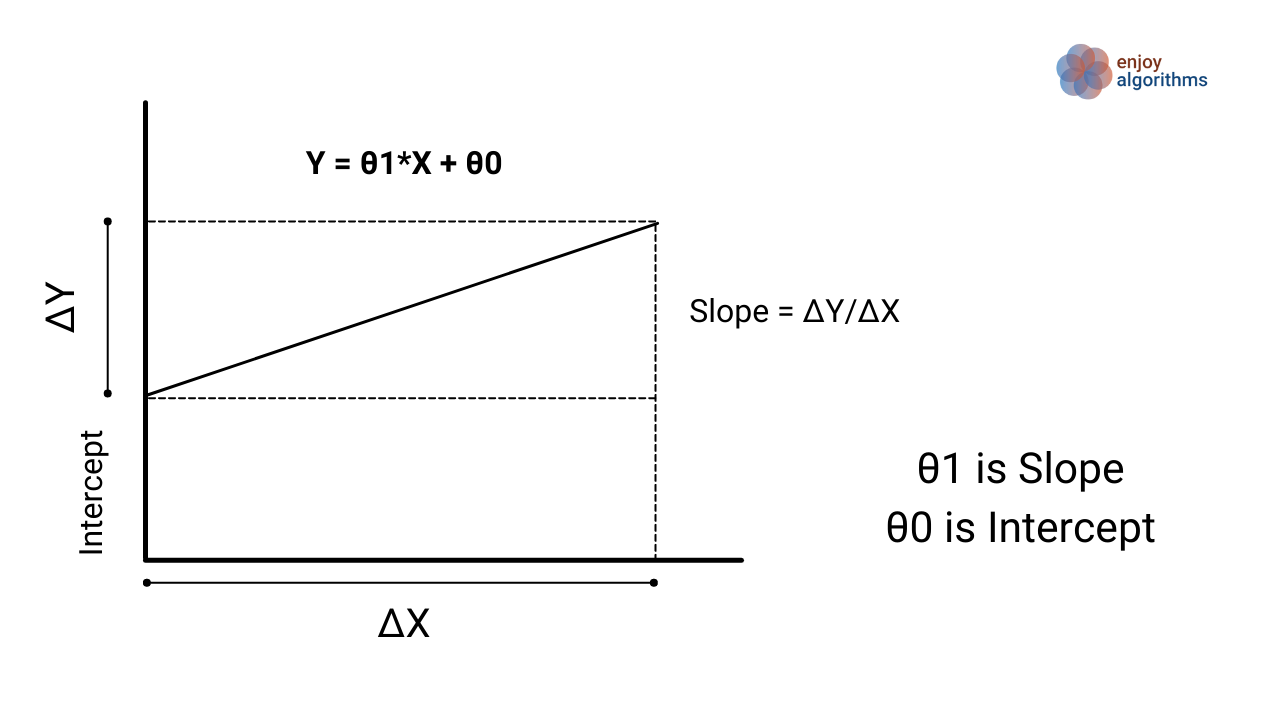Suppose we want to estimate a linear function from our input and output data coming from a linear equation Y = X + 1 (θ1 = 1 and θ0 = 1).

``````X = [0,1,2, ...., 50]
Y = [1,2,3, ...., 51]
``````

Correlating this case from our earlier scenario, now we have to find the values of both θ1 and θ0. For similarity, let’s assume the cost function is similar to the earlier case. Now we have to learn two different parameters that will affect the calculation of cost. To visualize it better, see the GIF below. There are three dimensions where we are trying to visualize the effect of parameters *θ1 and θ0* over the Cost function.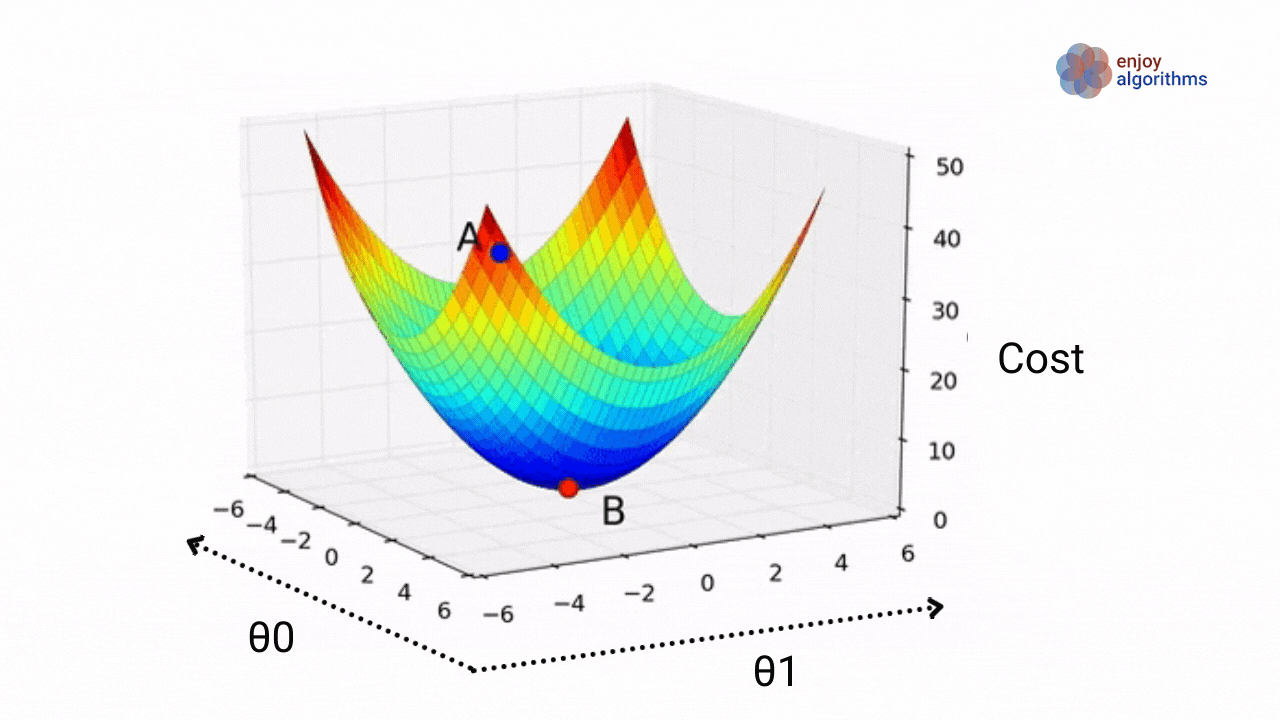Again in a step-wise manner,

Step1: The machine will select some random values of θ0 and θ1. (Let’s say θ0 = 6 and θ1 = -6) and based on this, it will calculate Y’, where Y’ = -6*X + 6. The position of point A in the above GIF.

Step 2: Now, the machine has actual values Y and estimated value Y’ based on a random guess of parameters. Using these, it will calculate the average error or cost function for all the input samples similar to the previous example.

Step 3: It will update the parameters θ0 and θ1 so that the cost function becomes as lesser as possible. In simple terms, it will try to reach point B in the above GIF.
Suppose after trying several combinations of θ0 and θ1; the machine was only able to find that θ0 = 0.9999 and θ1 = 1.0001 gives the minimum cost function. Somehow our machine missed checking the cost function for θ0 = 1 and θ1 = 1.

Step 4: The machine now has to store two parameters (θ0 = 0.9999 and θ1 = 1.0001) that it learned after trying various combinations of these parameters.

Machines generally store these values as weight and bias values.We can also summarize the above learning as “the machine has learned a Weight Vector θ1 and a Bias Vector θ0”.

Let's represent the above cost plot GIF as a contour plot.

A contour plot consisting many contour lines.The property of contour lines of two variable (in our case, it is θ1 and θ0) function (cost function) is that it has a constant value for all the points present on the same line.

In the image below, the value of the cost function for all the red-X points will be constant, and in the same manner, the cost function values for all the red-O will be constant.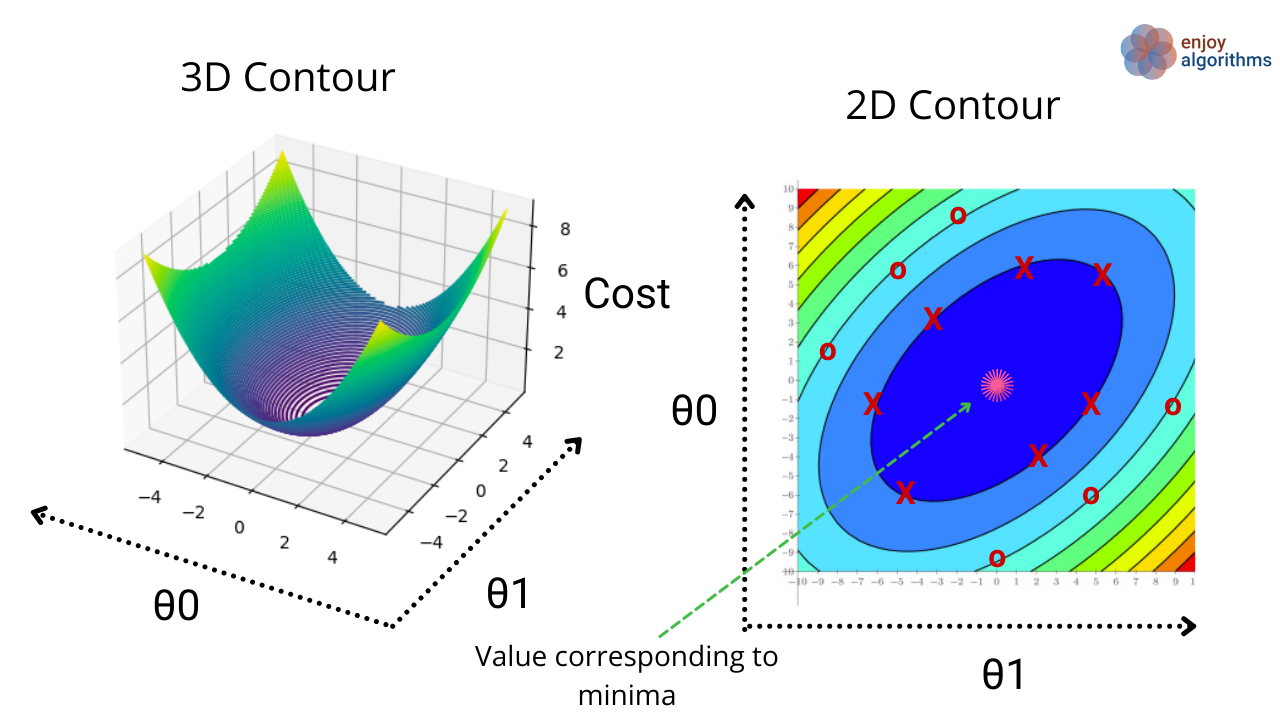If you observe the 3D contour image, the value of cost function at the innermost center is the minimum, and our objective is still the same, i.e., minimize the cost function. The machine will try to reach the pink star position by trying various values of θ1 and θ0 in the same fashion as we did in the earlier case of learning a single parameter.

Once our machine says that for θ1 = 1 and θ0 = 1, the cost function will be minimized, it will store these values as learned parameters and use them later for predictions.

This is all about how Machine Learns!!

### What if there are more than two parameters to learn?

There can be various scenarios where we need to learn a massive number of parameters. Let’s take one example. Suppose we have to learn a function of this format,Suppose we want to predict the house price. The price of a house depends on multiple factors, including the house's size, locality, market distance, water supply hours, and many more. These factors are represented via X1, X2, ..., Xn, and the importance of these factors is controlled by θ1, θ2, ..., θn, respectively.

We are trying to learn the mapping function for the house price, which is linear. To match this learning from the equation of Y = Weight*X + Bias, let's say X = [X1, X2, ..., Xn].T. Y is the price, and it will always be a 1x1 matrix of a house's price value.

Let's do some linear algebra and verify the matrix dimensions.

dimension(Y) = 1 x 1, dimension(X) = m x 1, so dimension(weight) must be of 1 x m. So, we have to learn a 1 x m dimension matrix entities along with the 1 x 1 bias value.

Similarly, Suppose X is not a number but an n-dimensional vector and Y is a (m x m) matrix,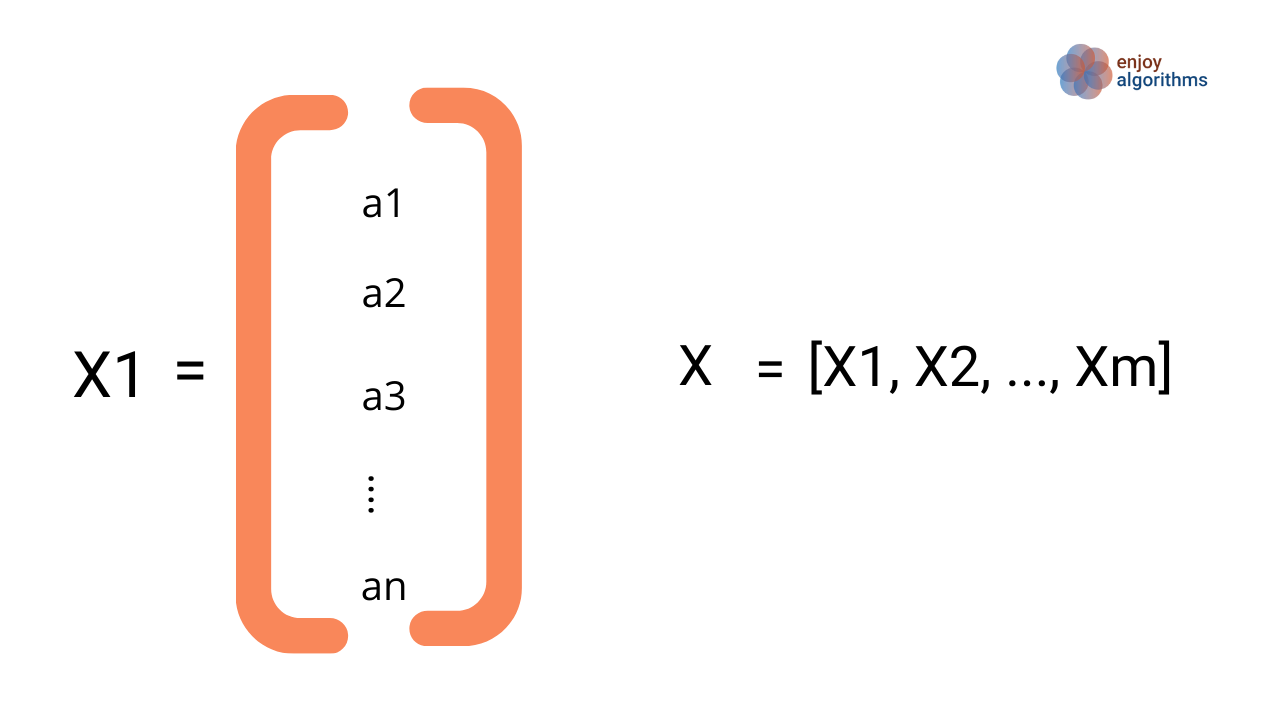If we correlate the above equation with *Y = WeightX + Bias**, we can represent the weight matrix as
dimension(Y) = m x m, dimension(X) = n x m and hence the weight matrix dimension would be, dimension(weight) = m x n, shown in the image below.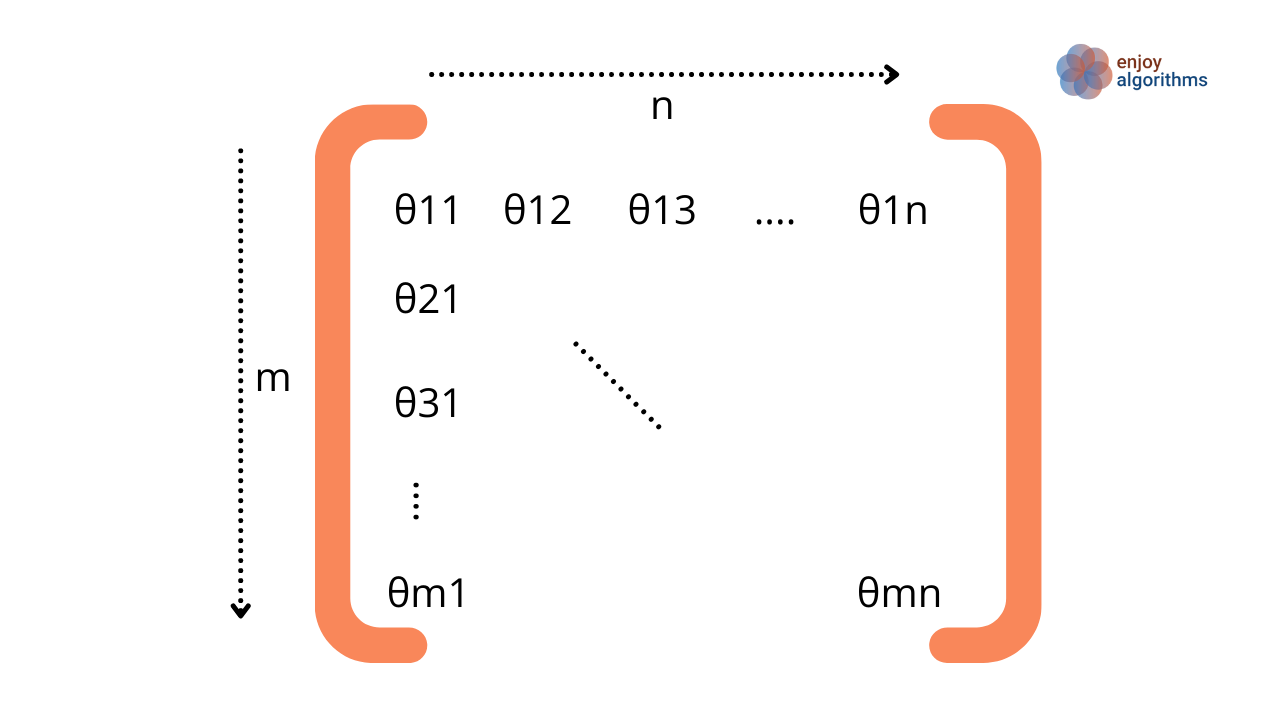So, machines will learn all these parameters (θ11, θ12, …, θmn) of the weight and the bias matrices.

### Possible Interview Questions

This article is about one of the most fundamental concepts in the field of Machine Learning. In Machine Learning interviews, it is always advisable to have in-depth knowledge about the basics rather than knowing the most complex algorithms. Some of the most frequent basic questions could be,

1. What is the Cost function of Machine Learning? Why do we define it?
2. If the machine selects random values and updates the parameter, what are the chances of hitting the minima of cost function?
3. How do machines store the learnings and utilize them for new input values?
4. What if Machines do not achieve the perfect minima?
5. What are contour plots?

### Conclusion

In this article, we developed a basic intuition behind the cost function involvement in machine learning. Through a basic example, we demonstrated the step-wise learning process of machines and analyzed how machine learning problems got converted into an optimization problem. In the last, we saw the contour plot of the two variables involved. We hope you enjoyed the article.

#### Enjoy Learning! Enjoy Thinking! Enjoy Algorithms!

Subscribe to get well-designed content on data structures and algorithms, machine learning, system design, oops, and mathematics. enjoy learning!

### We Welcome Doubts and Feedback!